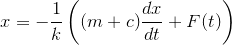Search IntMath
Close

# Euler’s Method and Runge-Kutta RK4

By Murray Bourne, 09 Mar 2015

I recently updated the interactive spring applet on this page (you'll see it about half way down):

Work by a variable force - spring appletThe upgrade (now javascript, rather than Flash) uses some interesting numerical solutions for a differential equation. It uses Runge-Kutta Order 4 Method. (Actually, my earlier Flash version also used Runge-Kutta method of Order 4, but it was a lot smoother than the javascript version. I'm still trying to improve it.)

The differential equation we need to solve for a spring-mass system is:where:

x is the position of a mass at the end of the spring at time t;

k is the spring constant;

c is the amount of damping (which slows the action of the spring); and

F is some external force, varying with time t.

The code works out a good approximation for the movement of the spring. In fact, this is how a lot of calculus solutions are obtained in the real world - numerically on computers, not algebraically like in math text books.

I didn't have anything on numerical solutions for differential equations in IntMath, so I added it just now. For introduction, I also wrote a section on Euler Method. Here are the 2 pages:

Euler's Method

Runge-Kutta (RK4) numerical solution for Differential Equations

I hope you find them useful.

Be the first to comment below.

### Comment Preview

HTML: You can use simple tags like <b>, <a href="...">, etc.

To enter math, you can can either:

1. Use simple calculator-like input in the following format (surround your math in backticks, or qq on tablet or phone):
a^2 = sqrt(b^2 + c^2)
(See more on ASCIIMath syntax); or
2. Use simple LaTeX in the following format. Surround your math with $$ and $$.
$$\int g dx = \sqrt{\frac{a}{b}}$$
(This is standard simple LaTeX.)

NOTE: You can mix both types of math entry in your comment.